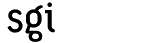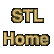# count_ifCategory: algorithms Component type: function

### Prototype

Count_if is an overloaded name: there are two count_if functions.
```template <class InputIterator, class Predicate>
iterator_traits<InputIterator>::difference_type
count_if(InputIterator first, InputIterator last, Predicate pred);

template <class InputIterator, class Predicate, class Size>
void count_if(InputIterator first, InputIterator last,
Predicate pred,
Size& n);
```

### Description

Count_if finds the number of elements in [first, last) that satisfy the predicate pred. More precisely, the first version of count_if returns the number of iterators i in [first, last) such that pred(*i) is true. The second version of count adds to n the number of iterators i in [first, last) such that pred(*i) is true.

The second version of count_if was the one defined in the original STL, and the first version is the one defined in the draft C++ standard; the definition was changed because the older interface was clumsy and error-prone. The older interface required the use of a temporary variable, which had to be initialized to 0 before the call to count_if.

Both interfaces are currently supported , for reasons of backward compatibility, but eventually the older version will be removed.

### Definition

Defined in the standard header algorithm, and in the nonstandard backward-compatibility header algo.h.

### Requirements on types

For the first version, which takes three arguments:
• InputIterator is a model of Input Iterator.
• Predicate is a model of Predicate.
• InputIterator's value type is convertible to Predicate's argument type.
For the second version, which takes four arguments:
• InputIterator is a model of Input Iterator.
• Predicate is a model of Predicate.
• Size is an integral type that can hold values of InputIterator's distance type.
• InputIterator's value type is convertible to Predicate's argument type.

### Preconditions

For the first version:
• [first, last) is a valid range.
For the second version:
• [first, last) is a valid range.
• n plus the number of elements that satisfy pred does not exceed the maximum value of type Size.

### Complexity

Linear. Exactly last - first applications of pred.

### Example

```int main() {
int A[] = { 2, 0, 4, 6, 0, 3, 1, -7 };
const int N = sizeof(A) / sizeof(int);

cout << "Number of even elements: "
<< count_if(A, A + N,
compose1(bind2nd(equal_to<int>(), 0),
bind2nd(modulus<int>(), 2)))
<< endl;
}
```

### Notes

 The new count interface uses the iterator_traits class, which relies on a C++ feature known as partial specialization. Many of today's compilers don't implement the complete standard; in particular, many compilers do not support partial specialization. If your compiler does not support partial specialization, then you will not be able to use the newer version of count, or any other STL components that involve iterator_traits.